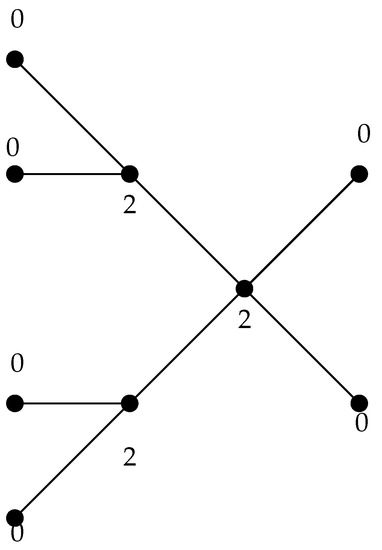Previous Article in Journal
A New Extension of the τ-Gauss Hypergeometric Function and Its Associated Properties
Open AccessArticle

# Strong Equality of Perfect Roman and Weak Roman Domination in Trees

byAbdollah Alhevaz 1,*,Mahsa Darkooti 1,Hadi Rahbani 1 andYilun Shang 21
Faculty of Mathematical Sciences, Shahrood University of Technology, P.O. Box 316-3619995161, Shahrood, Iran
2
Department of Computer and Information Sciences, Northumbria University, Newcastle NE1 8ST, UK
*
Author to whom correspondence should be addressed.
Mathematics 2019, 7(10), 997; https://doi.org/10.3390/math7100997
Received: 11 August 2019 / Revised: 6 October 2019 / Accepted: 16 October 2019 / Published: 21 October 2019
Let $G = ( V , E )$ be a graph and $f : V ⟶ { 0 , 1 , 2 }$ be a function. Given a vertex u with $f ( u ) = 0$ , if all neighbors of u have zero weights, then u is called undefended with respect to f. Furthermore, if every vertex u with $f ( u ) = 0$ has a neighbor v with $f ( v ) > 0$ and the function $f ′ : V ⟶ { 0 , 1 , 2 }$ with $f ′ ( u ) = 1$ , $f ′ ( v ) = f ( v ) − 1$ , $f ′ ( w ) = f ( w )$ if $w ∈ V ∖ { u , v }$ has no undefended vertex, then f is called a weak Roman dominating function. Also, the function f is a perfect Roman dominating function if every vertex u with $f ( u ) = 0$ is adjacent to exactly one vertex v for which $f ( v ) = 2$ . Let the weight of f be $w ( f ) = ∑ v ∈ V f ( v )$ . The weak (resp., perfect) Roman domination number, denoted by $γ r ( G )$ (resp., $γ R p ( G )$ ), is the minimum weight of the weak (resp., perfect) Roman dominating function in G. In this paper, we characterize those trees where the perfect Roman domination number strongly equals the weak Roman domination number, in the sense that each weak Roman dominating function of minimum weight is, at the same time, perfect Roman dominating. View Full-Text
Show FiguresFigure 1

MDPI and ACS Style

Alhevaz, A.; Darkooti, M.; Rahbani, H.; Shang, Y. Strong Equality of Perfect Roman and Weak Roman Domination in Trees. Mathematics 2019, 7, 997.